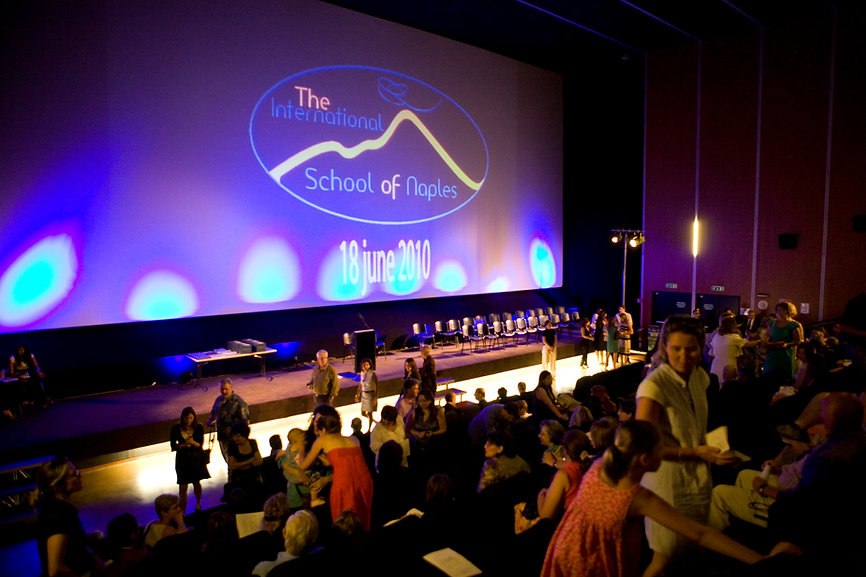Mathematics – Algebra 2

This course explores the mathematical concept of the function by extending students’ experiences with linear and quadratic relations. Students will investigate properties of discrete and continuous functions, including trigonometric and exponential functions.  Students will learn to represent functions numerically, algebraically, and graphically; solve problems involving applications of functions; investigate inverse functions; and develop facility in determining equivalent algebraic expressions. Students will also solve problems involving probability and statistics.  Students will reason mathematically and communicate their thinking as they solve multi-step problems.